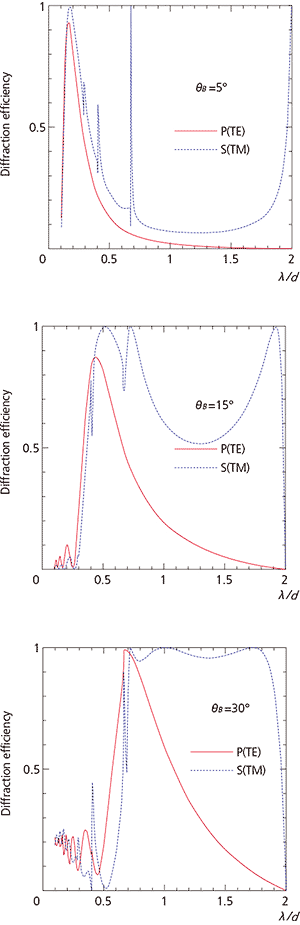Diffraction Efficiency & Relationship between Diffraction Efficiency and Polarization

Diffraction Efficiency
The diffraction efficiency is a value that expresses the extent to which energy can be obtained from diffracted light with respect to the energy of the incident light.
In general, there are two ways of expressing the diffraction efficiency, "absolute diffraction efficiency" and "relative diffraction efficiency." The absolute diffraction efficiency is the ratio of the diffracted light intensity, of a given order, to the incident light intensity, and the relative diffraction efficiency is obtained by dividing the absolute diffraction efficiency by the reflectance of the coating material. Shimadzu adopts the relative diffraction efficiency. The values given in catalogs correspond to λB(Litt) for plane gratings and λB for concave gratings.

Relationship between Diffraction Efficiency and Polarization
Because the grooves in a grating are all etched in one direction, the diffraction efficiency can vary significantly with the polarization of incident light.
With S-polarization (TM waves), where the direction of the grating grooves and the oscillation direction of electric field vectors is perpendicular, large fluctuations in the diffraction efficiency can be observed.
Also, a high diffraction efficiency is exhibited for long-wavelength regions. With P-polarization (TE waves), where the direction of the grating grooves and the oscillation direction of electric field vectors is parallel, there is not as much fluctuation as with Spolarization, and the diffraction efficiency describe a smooth curve that peaks at the blaze wavelength.
Fig. 7 shows the results of calculating the diffraction efficiency in the Littrow configuration for different polarizations. The horizontal axis represents the ratio of the wavelength λ to the grating period d, i.e., λ/d.
For larger blaze angles (grooves depth for sinusoidal gratings), scalar theory becomes less applicable and the diffraction efficiency varies greatly with the polarization.Fig. 7 Relationship between Diffraction Efficiency and Polarization